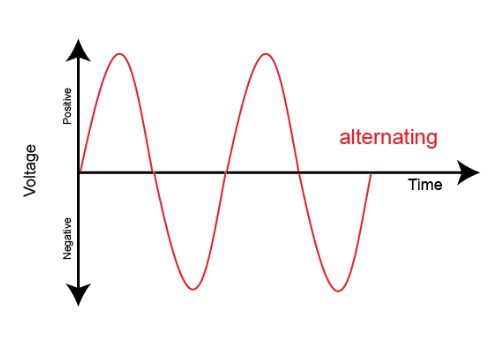# Introduction to Alternating Current## Introduction

AC stands for Alternating Current and is symbolised by “~”. This current alternates both its size (amplitude) and its direction.The figure for periods per second is called frequency and is stated in Hertz. 1 Hz = one period per second. The duration of one period is the period time, which is calculated as follows T=1/f

At a frequency of 50 Hz, the period time is 0.02 seconds. As opposed to DC voltage and direct current, which are characterised by having just one direction, AC voltage and alternating current are characterised by having more than one direction.Normally, the effective value is used. An alternating current of 1 A develops the same heat in a given resistor as a direct current of 1 A. Vectors are very useful with alternating currents and AC voltages. They clarify the connection between current, voltage and time. A vector is characterised by its length and direction of rotation. The positive direction of rotation is anti-clockwise. When a vector turns a full circle from its starting point, the vector peak runs 360°. The time of one revolution is identical to the period time of the sinusoidal curve. The vector speed per second is stated as angular speed and with the Greek letter ω. ω = 2×π×f. There are three types of AC loads.
If the load consists of coils with an iron core as in motors, the load is mainly inductive. In this case, the current is delayed in time in relation to the voltage.

The load can be capacitive. Here the current is ahead of the voltage in time. In the case of a purely resistive load, there is no displacement between current and voltage. The displacement between voltage and current is called the
angle of phase difference and the Greek letter Φ. Multiplying the current and voltage values which belong together
results in a power characteristic for the three types of load.The “pure” types of load are only theoretical values when AC circuits are described. A load is either inductive or capacitive.

### Power factor

The power factor λ is defined as the ratio between the active power and the apparent power. Often, this factor is also called cos ϕ; however, cos ϕ has only been defined for sinusoidal currents and voltages. In the case of non-linear loads such as the frequency converter, the load current is not sinusoidal. Consequently, a distinction must be made between cos ϕ and the power factor.P is the active power, IW is the active current, I and U are effective values. ϕ is the phase difference between current and voltage. If there is a purely sinusoidal current and voltage, cos ϕ thus corresponds to the relation between active power and apparent power.### 3-phase AC current

In a 3-phase voltage system, the voltages are displaced by 120° in relation to each other. The three phases are normally depicted in the same system of co-ordinates.The voltage between a phase conductor and the zero conductor is called phase voltage Uf and the voltage between two phases is called mains voltage UN. The ratio between UN and Uf is √3

### Star or delta connection

When a three-phase mains supply is subjected to a motor load, the motor winding are connected in star or delta formation.In star connection, each phase is connected to one end of the motor windings, while the other ends are short-circuited (star point). The voltage across the individual windings isFor the currents, the following applies: I1 = I2 = I3 = IN
In delta connection, the motor windings are connected in series.
Each connection point is connected to a phase.
The voltage across the individual windings is
UN = U1= U2 = U3
For the currents, the following applies